# The magnificient power of data exploration

First of all, we are all in aware of the power of data science come from the that of statistics. Therefore, take a close look some basic of data examination for a guide line.

Let’s we have a churn data set as below,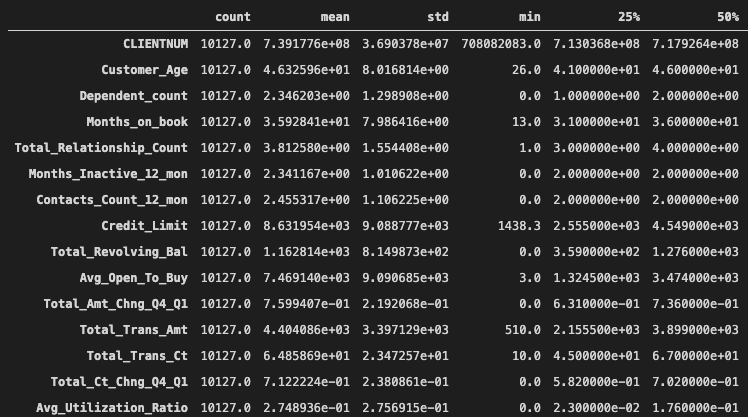Churndata set description.

Before diving in depth for estimation of output, everyone should seek for some basic statistical challanges such as missing data, outliers, correlations. I dont event mention about assumtions for given machine learning model.

One can see the outliers from the box plot visualisation of each variables.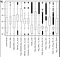Box-plots of each variables.

Normality distribution can be obtained from histogram plots as well.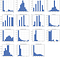Histogram plots of variables.

And correlations can be obtained with a piece of code.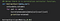Get correlation above 0.85.

Avg_Open_To_Buy and Credit_Limit have correlation of 0.986728

Total_Revolving_Bal and Credit_Limit have corelation of 0.986728

One can apply log transformation to a variable that is skewed more than 0.75. It is very easy to get these variables in pytonic way. By the way, although R is a statistical programming language most of the time, python can provide more elastic handling opportunities.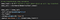Getting skewed variables.

And below the example of log transformation of a variable.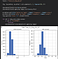Log transformation of variables.

After handling much of these hard and nasty works. Lets see if data examination phase is successful. Below a piece of code to make a knn classifier with churn dataset which is not been cleaned and examined.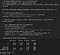Knn with uncleaned data.

If you can see, the accuracy score is 0.75. Lets try with the churn data that we have already examined and dealed with outliers, correlations etc.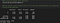Knn with cleaned data.

Wow, see how the accuracy score increase from 0.75 to 0.89. This kind of approach can save you much more time if you are not dealing with very complex models.

In fact, there is another perspective that I want to clarify in this reading. It is the meaning of probability density functions in terms of corelation. Lets see the pdf of a variable in churn data.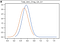pdf of Total_Amt_Chng for churned and not churned customer.

Can you see how indifferent this varaibles in terms of churned and unchurned customers. Therefore, this variable may not so well contribute to the estimation of customer churn. Lets take a look at another varaible.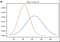pdf of Total_Trans_Ct for churned and not churned customer.

One can see the difference of churned and unchurned customers in terms of this variable. The thing is we can produce this kind of variables, which is called feature engineering. Lets see another variables that is produced from raw data.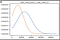Multiplying of two variables.

This is a new variable that can contribute to estimation power of our model. In order to measure the estimation power of a variable in terms of pdf, one can use area under curve approach as below,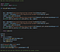AUC approach for pdf.

The output of this code is,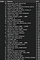Difference of AUC.

The difference of AUC is another perspective of data examination that can be utilized in data examination phase of machine learning approaches.

More the data we have, more the understanding we get.

## More from ulkuozturk

More the data we have, more the understanding we get.